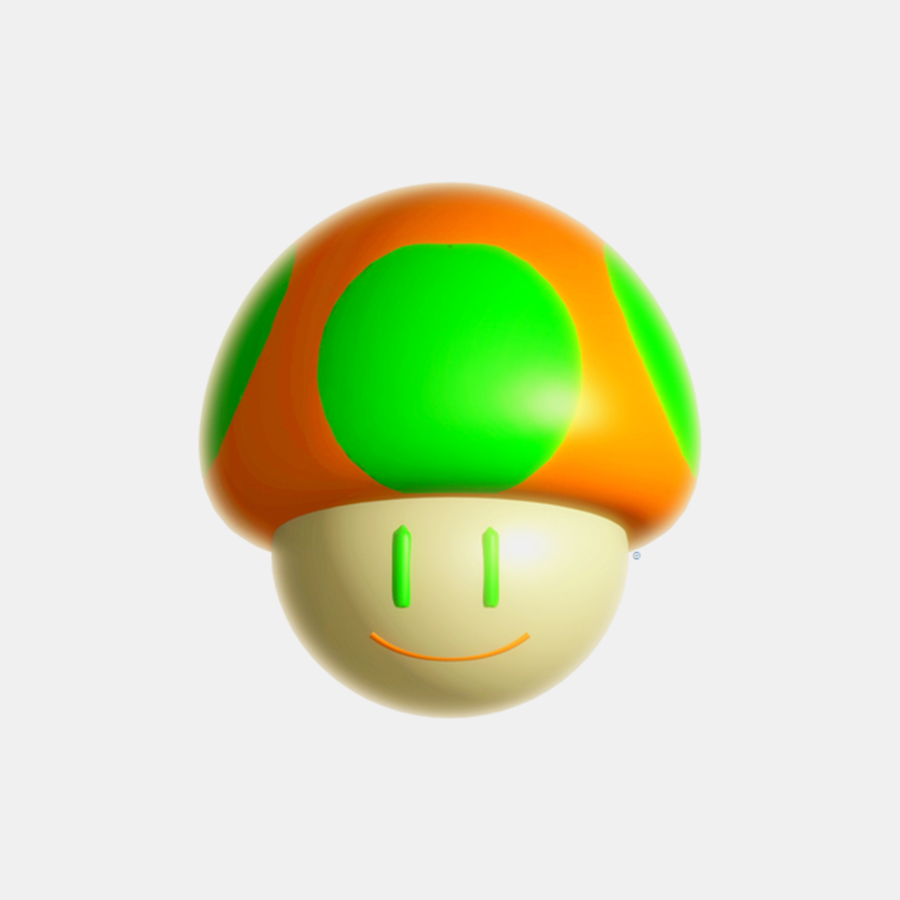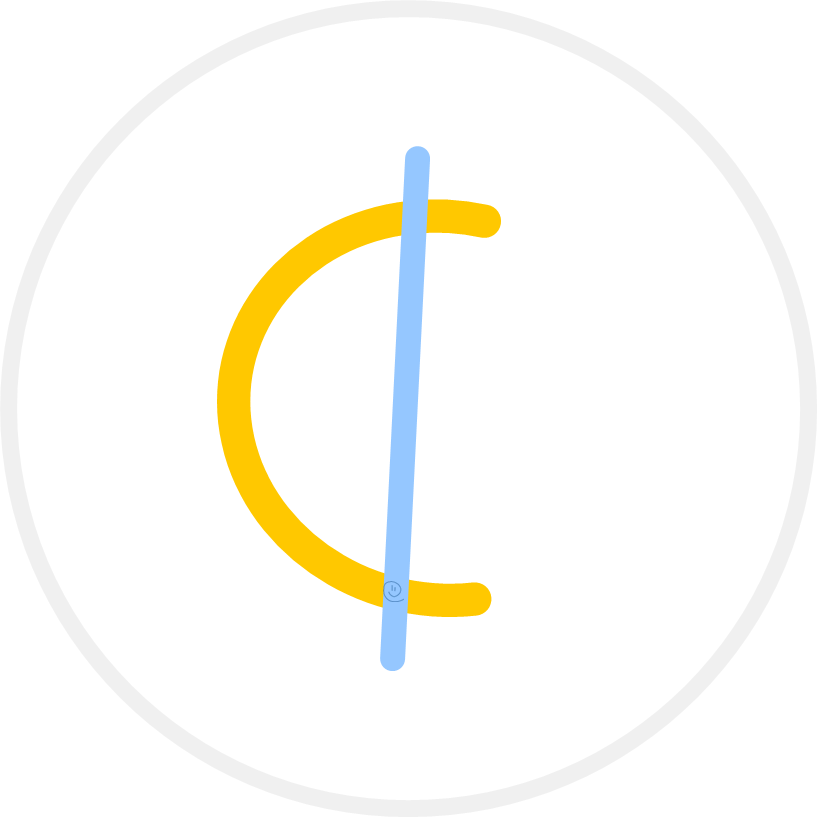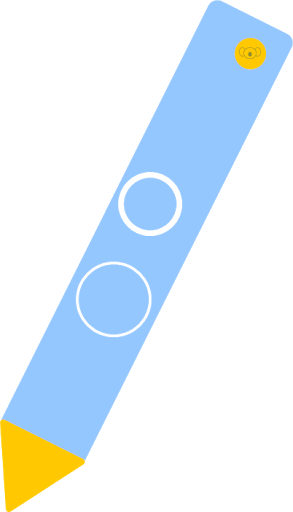Showing posts with label algorithm. Show all posts
Showing posts with label algorithm. Show all posts

### +strategy

Within the context of gameplay, a strategy is a genetic algorithm/heuristic* for solving a puzzle🧩 and predicting/identifying (walk) patterns from random coil.** `decryption↦heuristic, algorithm↦encryption` Strategies are logged into behavioral cloning.Our strategic goals are to find and exploit lower bounds on (the number of) plays it takes to arrive at a zero-bubble. (see touch-and-go, improvise, juke notation, UUhistlegrass)
/// Strategies are typically non-brute force, and not `runtime`-dependent.

### +quotient normalization

Also known as '', quotient* normalization (qn), or, plainly, normalization, is the regulation of juke tax so that the (a limiting function) is load-balanced when perturbations to the router are overweighted [eg. excess tokens].** of StewartThe eventuality that - because the jukebox can purse^ but not bank - a quotient normalization implicates juking as complexity-agnostic (ie. is most fit) at inflection points [instances where ], would bias the Pink program to tipping (concavity or convexity).^^ `Coupon partitioning` is a `function` of `credit`; whereas `taxation` is a `debit` `function`. (see quantumquotient, penny fret, 🧑🏿lnq's bubblegum, 🧑🏿lnq's walk, thread, parimutuel, Funday)/// Quotient normalization is a preliminary step in `parimutuel` auctioning.

### +The Origamic Symphony🎶The Origamic Symphony™℗ (Q) is the totality of Egglepple('s) movements (ie. keys in sequence).Resting atop the hypothesis that every brane arrangement is (geometrically) convertible, solvable, and scorable, Q♯ is responsive to the calculus of Egglepple resonances (the program of the portfolio imagined as frets pertinent to stereotyping EGP)."There is this beautiful symphony playing - everywhere, all the time."^ - 🧑🏿Link Starbureiy
^^ Symphonic evidence is its subject (UUelcome).

The symphony treats the entire portfolio as a (single loopstring*) path integral (line) subject to integration (including integration-by-parts [the twisting of pencils]) and derivation.** A continuous `function`. -- All walks are integrated in twistorspace, and output some stew derivative. (see UUelcome, quantumquotient, UUhistlegrass, 🧑🏿lnq's Starting Five, Juke Lemma)/// +The Origamic Symphony is the subject of 🧑🏿lnq's recital.
+Here "origami" specifies "p-`brane` (`membrane`/`frame`) folding". Namely, it is the `twisting` of `measures` (length) between the Planck and nano `data`.

### +mathematics

Mathematics is an ambiguous way to prove or disprove ideal behaviors of objects.
/// An '`open` story of counting', it must be said that mathematical methodology is a cumulative effort (ie. rigorously `built` atop previous checks).

As libraries* for synthesizing functions, mathematicians use its models to formulate (computability) theories/theorems/identities/errata.** We acknowledge the so-called 'Big Five (5)' active areas in mathematics to be [1-5 labeled alphabetically]: algebra , analysis , arithmetic , geometry , and music . As far as I am concerned, the basis of math is `juking`. (see also mathemusic, mathletics, recreational mathematics, mathematical model, Mathilda, 📓So, you want to be a mathematician?, The Mathemagician, Opus Solve, physics, information science, game, cryptosport, 🧩puzzle)

"The essence of mathematics is not proof, but conjecture." - 🧑🏿Link Starbureiy

/// +There are three (3) `classes` of mathematics: pure, applied, and recreational (pure,applied ∈ recreational). This `definition` covers all three (3), and may be referred to as polymathematics. A mathematician is someone who advances `classical` mathematics.
+In my line of work, I think of so-called 'recreational mathematics' as a `type` of (among other considerations) reverse engineering, where we are re-creating known structures/models for study and understanding. As an example, in `🧩puzzle solving`, I may take a known and `solved` (from means other than `juking`) macromolecule (eg. protein), and `juke` that `fibor` so that it can identified+`databased`. (see also Pajamas)
+Mathematics is a `type` of low-technology, as well as being its own industry.

----------
#LEGEND
math.AG (algebraic geometry), math.AT (algebraic topology), math.AP (analysis of partial differential equations), math.CT (category theory), math.CA (classical analysis and ordinary differential equations), math.CO (combinatorics), math.AC (commutative algebra), math.CV (complex variables), math.DG (differential geometry), math.DS (dynamical systems), math.FA (functional analysis), math.GM (general mathematics), math.GN (general topology), math.GT (geometric topology), math.GR (group theory), math.HO (history and overview), math.IT (information theory), math.KT (k-theory and homology), math.LO (logic), math.MP (mathematical physics), math.MG (metic geometry), math.NT (number theory), math.NA (numerical analysis), math.OA (operator algebras), math.OC (optimization and control), math.PR (probability), math.QA (quantum algebra), math.RT (representation theory), math.RA (rings and algebras), math.SP (spectral theory), math.ST (statistics theory), math.SG (symplectic geometry)

### +mathemusic

Mathemusic is the functional conflation of brane classes defined as 'an incorporation of keys and timing to perturb a sinusoidal, whose resultant is a γ-proof'.

This tends more towards being a supermathematical exercise grounded in the principles of music theory. However, it is practically expressing (with libretti) statistics in the framework of 🎵music (even sans sonically). Cryptologically, it is the algorithmic direction of variation*.** `Solutions` have `melodic` texture.

When we speak of mathemusic, we are generally doing so in the context of The Origamic Symphony🎶/Egglepple. (see ¢ent, mathletics, 🧑🏿lnq's Starting Five, 3-rex, fibor, UUe, juke notation)

"All I hear is mathematics. All I see is music." - 🧑🏿Link Starbureiy/// Contemporary mathemusic was originally formulated by 🧑🏿Link Starbureiy.🤓

### +pink poemThe Pink program* is a class of algorithms for proving the quantumquotient.** `Calls+declarations` for `string` improvisation.

A pink poem ('pink') optimizes juking ludology [ie. (openingmiddlegameendgame)] for predicting Egglepple's imagery from its preimagery.++Each poem (a genetic algorithm / genetic algebra) is its own complete verse leading to fibor . (see 🧑🏿lnq's Starting Five, UUhistlegrass, touch-and-go, opera, patch, cassette, UUelcome, QQ)/// +It is `called` a "pink poem" just because Link Starbureiy liked how that color [RGB:255,0,100] (I call it watermelon🍉) looked on the design of the UUelcome Matte (and for the matching letter p's😉).
+The Pink program is the recital (concert) `program`, and a pink poem is essentially a `program` note.
+Pinks share a common `gameplay` directive with 🧑🏿lnq's bubblegum.

### +γ-proof

A yesegalo proof, or γ-proof (or just proof), is a mapping some preimage to its image. Proofing substantiates a fibor, thereby providing (its) identity clarification.!!! While also helping us `draw` up better heuristics. The opposite of a proof is an error. Each proof involves patching two (2) genera in T: yesegalo↔🍄meshroom.^^^ A `framework` for `conduction`: does a `ballet` groove with(in) the Symphony🎶?γ-proofing tasks: determine the efficiency of an EGP encryption [ie. solve the given puzzle🧩; is the resulting fibor economical (fit within the handicap)|(u,u)=0|?].The proof itself is scored* as a stereotype (sequenced shapeframe) in some overall random walk.** Proofs of `juke` `input` are published (to the ledger) in `juke notation`.

The end🏁 of a proof (solution) should result in the closing🔒 of a string (eg. fibor), ideally a zero-bubble. We endeavor to find the most secure🔐 proofs by improving upon the bounds on classes of twistors. Doing so aids our MONEY-making. (see juke notation, mathematical proof, proof.m)/// As with the traditional `printing` press, the primary reason for `proofing` is to provide confirmation/verification that a job (done) is accurate. Doing so `draws` a consensus (from independent, third-party sources -- read: `jukers`) for the creation of a new standard. It is important to note that `proofs` are unique (ie. duplication is forbidden on the circuit). All `proofs` are published to the ledger.
Function map: loopstringγ-proofMONEYquantumquotient

### +play

Per some ludology, a play is a legal move(ment) within a game. As a fold progression (eg. a scribble variant when juking Egglepple), each play [a finite step towards some goal] is an expression of some strategy (mathemusically, the duration of a coverage). (see game, gameplay, pink poem)The number of unique plays (p) can be computed from the superalgebraic resultant: p:≡Sg, where S is some integer value between 1 - 676, and g equals the amount of circuiting* twistorspace (ie. degrees of freedom [base] to the power [exponent] of residue bond angles).** Asymptotic tempo `values` completing the loop./// +The formula is in direct correlation to spin-statistics. So, for example, when b is odd, say b=11, then c(=32) is an integer-spin (egg). When b is even, say b=20, then c(=724.0+) is a half-integer-spin (epp). The rational portion (floating point) of half-integer statistics contributes to seigniorage. Obviously, any negative `value` for b will `yield` an epp. (see `juke tax`)
+Yes, there are twenty-six (26) integer `values` here; we denote "0" as (0-,0+) → having both negative(-) and positive(+) polarity in `twistorspace`. For instance, -12 ... (0-,0+) ... +12 = . p is an upperbound for points on a curve, and so its number line must include 0 (hence 0 through 25). The minima (cover-0) is 0.0001220703125 and the maxima (cover-25) is 4,096.

Plays (fugue protocols) are implemented as jukes (written in juke notation). They are executed [run] by the call action, and will typically end once the strategy is exhausted. There are three (3) possible outcomes of a play: loss (of advantage), futility (no advancement), or gain (advantage). (see player, playoff)

### +juke notation

Juke notation (jn, rhetorically called a verse) is the instrument-agnostic, language-independent, easy-to-decipher verbalization of some juke assignment as meant for proof. In signing, the alphanumeric notation is essentially low-level symbolic "play code"*; an algorithm devised to instruct the jukebox on how, when, and what to type in stereo [stew choreography].** Referred to as a track (for tracing some `token`).

The Stewdio is the designated instrument for generating, reading, routing, and interpreting juke notation. (See hologram, touch-and-go, γ-proof, thread, jukespace. Compare genetic code, musical notation)/// Juke notation was devised by 🧑🏿Link Starbureiy.🤓

### +hologram(see sticker, stamp, mesh, touch-and-go, juke notation, fibor, hash, mathematical model)

### +opera

An opera is the program (of opus numbers) of a libretto. (see opus, developus, opera ludo, hash opera, #opéra-ballet, ballet, #libretto, γ-proof)/// To me, an opera represents the 'flow of a `proof`'. It can also be a `runtime`. The works highlight `puzzles🧩` for identification.

### +MONEY algorithm

``` procedure // make MONEY1. begin juke2. when cap = yield 3. then your coupon collects a dividend(¢)😊 4. else { no fitness } improvise5. or echo {juke again}😉```